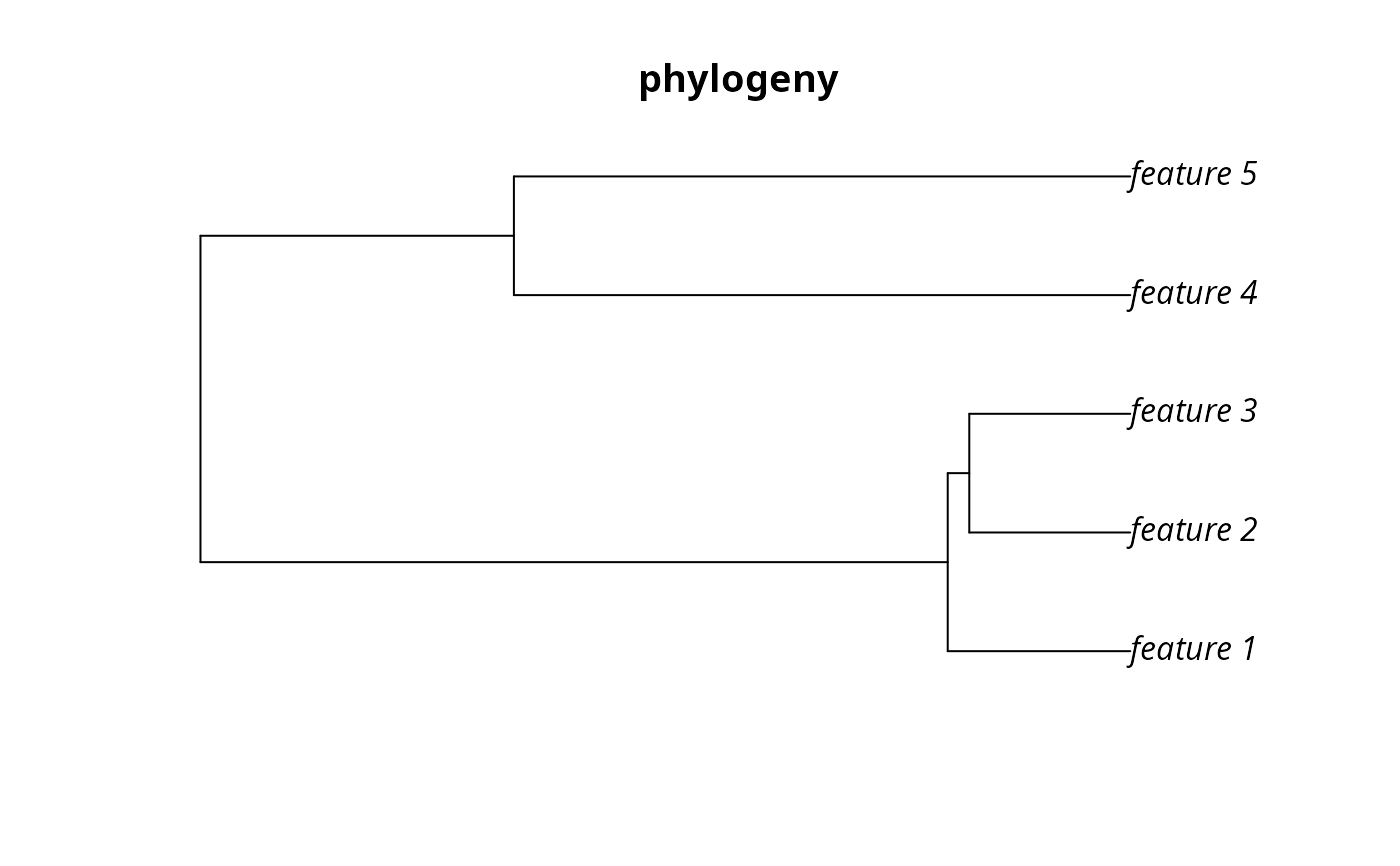Phylogenetic trees depict the evolutionary relationships between different species. Each branch in a phylogenetic tree represents a period of evolutionary history. Species that are connected to the same branch both share that same period of evolutionary history. This function creates a matrix that shows which species are connected with branch. In other words, it creates a matrix that shows which periods of evolutionary history each species have experienced.

branch_matrix(x)

# S3 method for default
branch_matrix(x)

# S3 method for phylo
branch_matrix(x)

## Arguments

x ape::phylo() tree object.

## Value

dgCMatrix sparse matrix object. Each row corresponds to a different species. Each column corresponds to a different branch. Species that inherit from a given branch are denoted with a one.

## Examples

# load data
data(sim_phylogeny)

# generate species by branch matrix
m <- branch_matrix(sim_phylogeny)

# plot data
# \dontrun{
par(mfrow = c(1,2))
plot(sim_phylogeny, main = "phylogeny")
plot(raster(as.matrix(m)), main = "branch matrix", axes = FALSE,
box = FALSE)# }